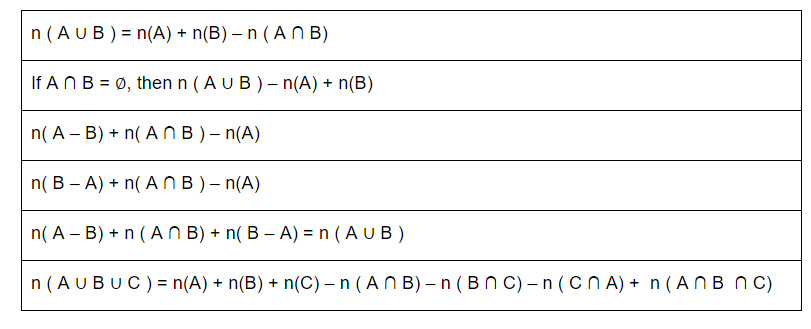# What is a Set?

In Maths, sets are a collection of well-defined objects or elements. A set is represented by a capital letter symbol and the number of elements in the finite set is represented as the cardinal number of a set in a curly bracket {…}. For example, set A is a collection of all the natural numbers, such as A = {1,2,3,4,5,6,7,8,…..∞}. Also, check sets here.

Sets can be represented in three forms:

1. Roster Form: Example- Set of even numbers less than 8={2,4,6}
2. Statement Form: Example-A = {Set of Odd numbers less than 9}
3. Set Builder Form: Example: A = {x: x=2n, n ∈ N and 1 ≤ n ≤ 4}

## What are the types of Sets?

A set has many types, such as;

1. Empty Set or Null set: It has no element present in it.Example: A = {} is a null set.
2. Finite Set: It has a limited number of elements.Example: A = {1,2,3,4}
3. Infinite Set: It has an infinite number of elements.Example: A = {x: x is the set of all whole numbers}
4. Equal Set: Two sets which have the same members.Example: A = {1,2,5} and B={2,5,1}: Set A = Set B
5. Subsets: A set ‘A’ is said to be a subset of B if each element of A is also an element of B.Example: A={1,2}, B={1,2,3,4}, then A ⊆ B
6. Universal Set: A set which consists of all elements of other sets present in a Venn diagram.Example: A={1,2}, B={2,3}, The universal set here will be, U = {1, 2,3}

## Formulas For Sets

Let us consider A, B and C are three sets.## Questions and Solutions on Sets

1. What is a Power set? Define with example.

Answer: In set theory, the power set of a set A is defined as the set of all subsets of the Set A including the Set itself and the null or empty set.

For Example: A = {1, 3, 5}

Power set of A, P(A) = {{}, {1}, {3}, {5}, {1,3}, {3,5}, {1,5}, {1,3,5}}

2. Let A and B be two finite sets such that n(A) = 25, n(B) = 20 and n(A ∪ B) = 30, find n(A ∩ B).

Solution: By the formula n(A ∪ B) = n(A) + n(B) – n(A ∩ B)

Hence, n(A ∩ B) = n(A) + n(B) – n(A ∪ B)

= 25 + 20 – 30

= 45 – 30

= 15

3. If n(A – B) = 30, n(A ∪ B) = 65 and n(A ∩ B) = 22, then find n(B).

Solution: By the formula, we know;

n(A ∪ B) = n(A – B) + n(A ∩ B) + n(B – A)

65 = 30 + 22 + n(B – A)

65 = 52 + n(B – A)

n(B – A) = 65 – 52

n(B – A) = 7

Now n(B) = n(A ∩ B) + n(B – A)

= 22 + 7

= 15

## Video Lesson on What are Sets